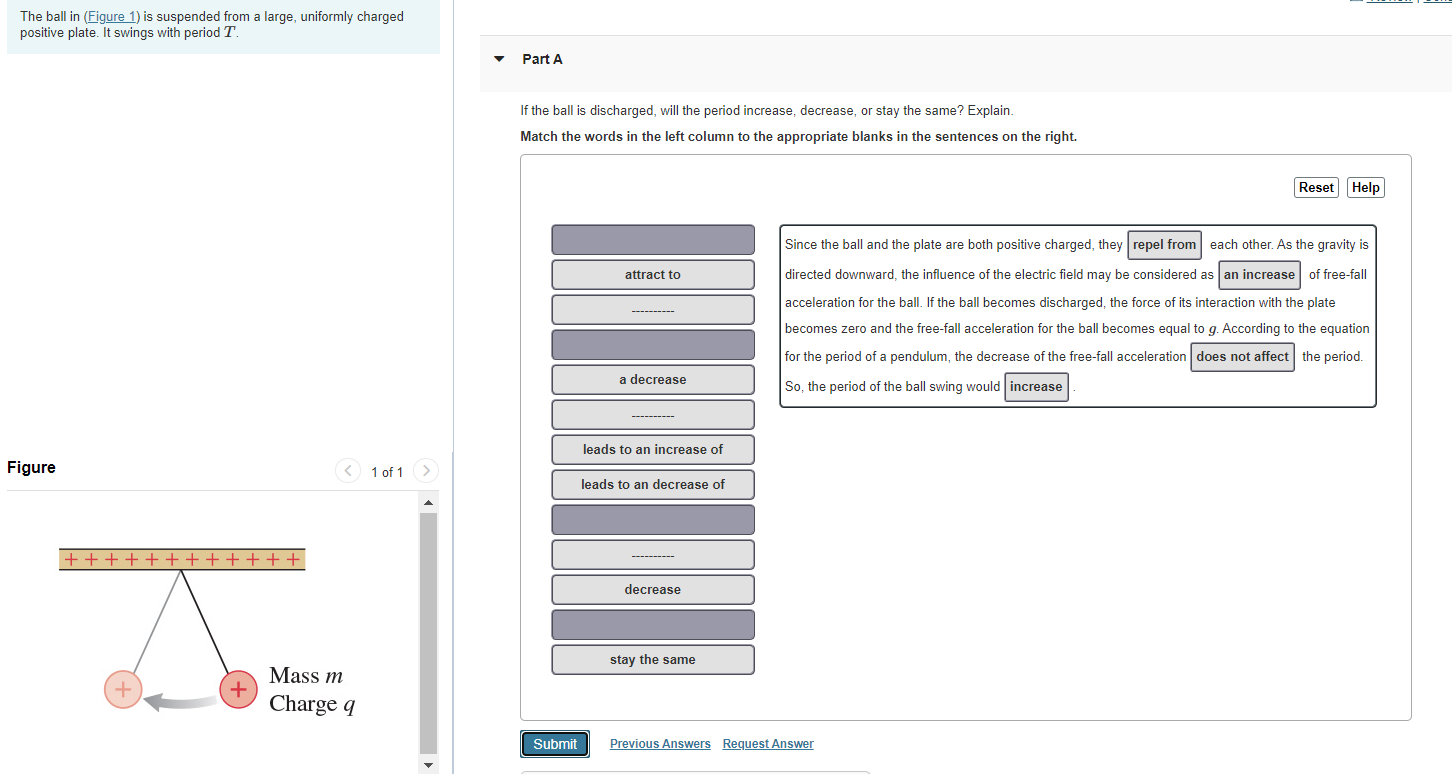# Question Solved1 AnswerThe ball in (Figure 1) is suspended from a large, uniformly charged positive plate. It swings with period T. Part A If the ball is discharged, will the period increase, decrease, or stay the same? Explain. Match the words in the left column to the appropriate blanks in the sentences on the right. Reset Help attract to Since the ball and the plate are both positive charged, they repel from each other. As the gravity is directed downward, the influence of the electric field may be considered as an increase of free-fall acceleration for the ball. If the ball becomes discharged, the force of its interaction with the plate becomes zero and the free-fall acceleration for the ball becomes equal to g. According to the equation for the period of a pendulum, the decrease of the free-fall acceleration does not affect the period. So, the period of the ball swing would increase a decrease --------- leads to an increase of Figure < 1 of 1 leads to an decrease of tttttttttttt decrease stay the same Mass m Charge a Submit Previous Answers Request AnswerTranscribed Image Text: The ball in (Figure 1) is suspended from a large, uniformly charged positive plate. It swings with period T. Part A If the ball is discharged, will the period increase, decrease, or stay the same? Explain. Match the words in the left column to the appropriate blanks in the sentences on the right. Reset Help attract to Since the ball and the plate are both positive charged, they repel from each other. As the gravity is directed downward, the influence of the electric field may be considered as an increase of free-fall acceleration for the ball. If the ball becomes discharged, the force of its interaction with the plate becomes zero and the free-fall acceleration for the ball becomes equal to g. According to the equation for the period of a pendulum, the decrease of the free-fall acceleration does not affect the period. So, the period of the ball swing would increase a decrease --------- leads to an increase of Figure < 1 of 1 leads to an decrease of tttttttttttt decrease stay the same Mass m Charge a Submit Previous Answers Request Answer
More
Transcribed Image Text: The ball in (Figure 1) is suspended from a large, uniformly charged positive plate. It swings with period T. Part A If the ball is discharged, will the period increase, decrease, or stay the same? Explain. Match the words in the left column to the appropriate blanks in the sentences on the right. Reset Help attract to Since the ball and the plate are both positive charged, they repel from each other. As the gravity is directed downward, the influence of the electric field may be considered as an increase of free-fall acceleration for the ball. If the ball becomes discharged, the force of its interaction with the plate becomes zero and the free-fall acceleration for the ball becomes equal to g. According to the equation for the period of a pendulum, the decrease of the free-fall acceleration does not affect the period. So, the period of the ball swing would increase a decrease --------- leads to an increase of Figure < 1 of 1 leads to an decrease of tttttttttttt decrease stay the same Mass m Charge a Submit Previous Answers Request Answer